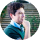# Variable Interest RateRoman Danaev

25 May 2023

## Meaning and Definition

A variable interest rate, also known as a floating interest rate, is an interest rate that can change over time. Unlike a fixed interest rate, which remains constant for the duration of the loan, a variable interest rate can fluctuate based on changes in an underlying reference rate, such as the base rate set by the Bank of England or the market conditions.

## Why it is important to know

Understanding the concept of a variable interest rate is important for borrowers as it introduces an element of uncertainty into the cost of borrowing. Changes in the underlying reference rate or market conditions can lead to fluctuations in the interest rate, which in turn affects the monthly payments and the overall cost of the loan. Borrowers need to be aware of this potential variability and consider their ability to absorb changes in interest rates when choosing a car finance agreement with a variable interest rate.

## Example in car finance

Suppose a borrower obtains a car finance agreement with a variable interest rate linked to the baserate set by the Bank of England. Initially, the interest rate is set at 3%. However, if the base rate increases, the interest rate on the car finance agreement may also increase. For example, if the base rate rises to 1%, the interest rate on the car finance agreement may increase to 4%. As a result, the borrower's monthly payments would increase, impacting the affordability of the loan. Conversely, if the base rate decreases, the interest rate on the car finance agreement may decrease, potentially leading to lower monthly payments. The variability of the interest rate in a variable interest rate car finance agreement introduces an element of uncertainty for borrowers, and they need to consider this when deciding on their preferred type of interest rate structure.

## Car finance calculator

Must be between £3,000 to £50,000
24month
36month
48month
60month